# AP Calculus AB Practice Test 30

### Test Information9 questions24 minutes

Calculator Allowed

1. If y = f (x2) and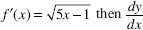is equal to

2. Find the area of the first quadrant region bounded by y = x2, y = cos (x), and the y-axis.

3. If the substitution x = 2t + 1 is used, which of the following is equivalent to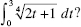4. At noon, an experimenter has 50 grams of a radioactive isotope. At noon 9 days lateronly 45 grams remain. To the nearest day, how many days after the experiment startedwill there be only 20 grams?

5. A 26-foot ladder leans against a building so that its foot moves away from the buildingat the rate of 3 feet per second. When the foot of the ladder is 10 feet from thebuilding, the top is moving down at the rate of r feet per second, where r is

6. If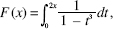then F (x) =

7.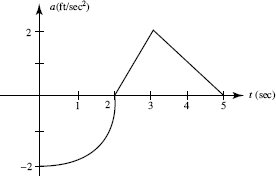The graph above shows an object's acceleration (in ft/sec2). It consists of a quarter-circle and two line segments. If the object was at restat t = 5 seconds, what was its initial velocity?

8. Water is leaking from a tank at the rate of R(t) = 5 arc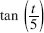gallons per hour, where t is the number of hours since the leak began. How many gallons will leak out duringthe first day?

9. Find the y-intercept of the line tangent to y = (x3 - 4x2 + 8)ecos x2 at x = 2.